## 4 Ways to Factor Trinomials: Algebra

Greeting math peeps and welcome to MathSux! In this post, we are going to go over 4 ways to Factor Trinomials and get the same answer, including, (1) Quadratic Formula (2) Product/Sum, (3) Completing the Square, and (4) Graphing on a Calculator.  If you’re looking for more don’t forget to check out the video and practice questions below.  Happy Calculating! 🙂

Also, if need a review on Factor by Grouping or Difference of Two Squares (DOTS) check out the hyperlinks here!

*If you haven’t done so, check out the video that goes over this exact problem, and don’t forget to subscribe!

We will take this step by step, showing 4 ways to factor trinomials, getting the same answer each and every time! Let’s get to it!

## 4 Ways to Factor Trinomials

____________________________________________________________________

## (2) Product/Sum: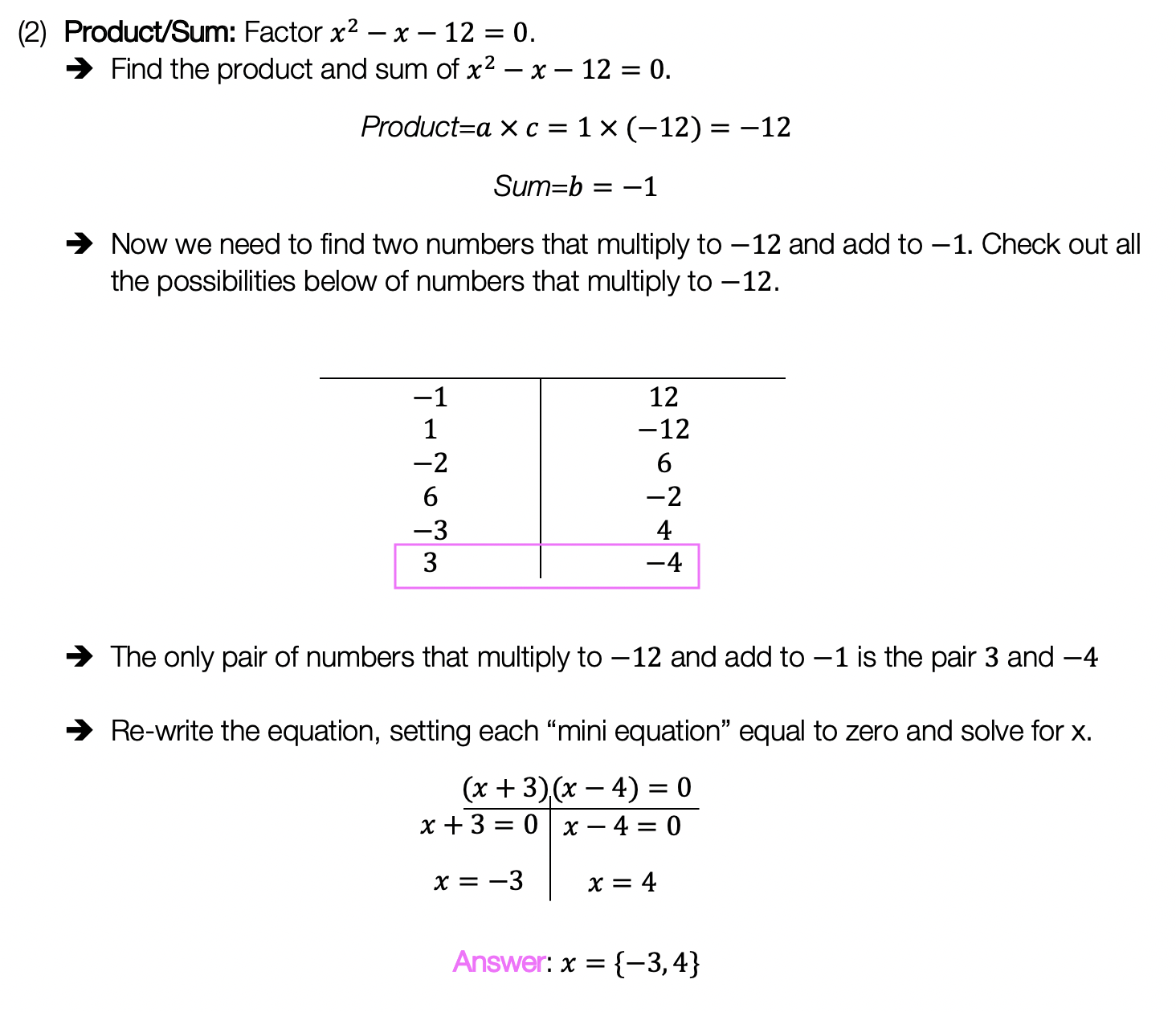____________________________________________________________________

## (3) Completing the Square: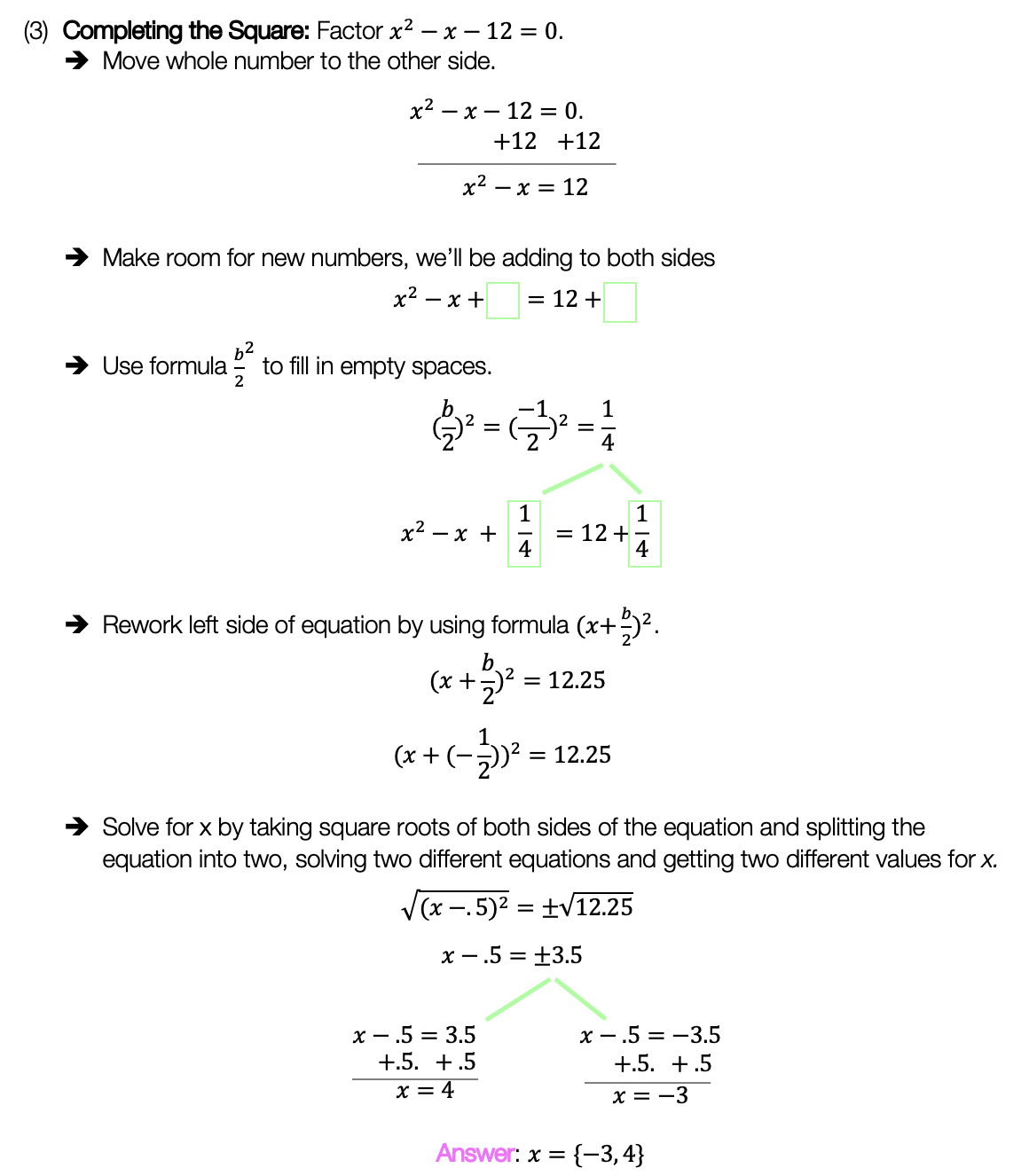____________________________________________________________________

## (4) Graph:

Choose the factoring method that works best for you and try the practice problems on your own below!

Practice Questions:

Solutions:

Want a review of all the different factoring methods out there?  Check out the ones left out here (DOTS and GCF) and happy calculating! 🙂

For even more ways to factor quadratic equations, check out How to factor by Grouping here! 🙂

Looking for more on Quadratic Equations and functions? Check out the following Related posts!

Factoring Review

Factor by Grouping

Completing the Square

The Discriminant

Is it a Function?

Imaginary and Complex Numbers

Quadratic Equations with 2 Imaginary Solutions

Focus and Directrix of a Parabola

Also, if you want more Mathsux?  Don’t forget to check out our Youtube channel and more below! If you have any questions, please don’t hesitate to comment below. Happy Calculating! 🙂

## Median of a Trapezoid Theorem: Geometry

Hi everyone and welcome to Math Sux! In this post, we are going to look at how to use and applythe median of a trapezoid theorem. Thankfully, it is not a scary formula, and one we can easily master with a dose of algebra. The only hard part remaining, is remembering this thing! Take a look below to see a step by step tutorial on how to use the median of a trapezoid theorem and check out the practice questions at the end of this post to truly master the topic. Happy calculating! 🙂

*If you haven’t done so, check out the video that goes over this exact problem, also please don’t forget to subscribe!

Step 1:  Let’s apply the Median of a Trapezoid Theorem to this question!  A little rusty?  No problem, check out the Theorem below.

Median of a Trapezoid Theorem: The median of a trapezoid is equal to the sum of both bases.Step 2: Now that we found the value of x , we can plug it back into the equation for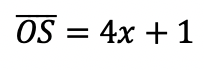median,  to find the value of median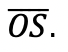Want more practice?  Your wish is my command! Check out the practice problems below:

Practice Questions:

1.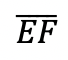is the median of trapezoid ABCDEF, find the value of the median, given the following:2.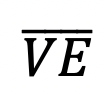is the median of trapezoid ACTIVE, find the value of the median, given the following:3.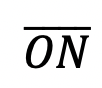is the median of  trapezoid DRAGON, find the value of the median, given the following:

4.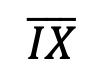is the median of trapezoid MATRIX, find the value of the median, given the following:

Solutions:

Need more of an explanation?  Check out the detailedand practice problems. Happy calculating! 🙂

## COVID-19: What does #FlattenTheCurve even mean?

COVID-19: What does #FlattenTheCurve even mean? If you are a human on Earth, then I’m sure you’ve heard about the coronavirus and are currently social distancing. Here in NYC, I’m quarantining like everyone else and listening to all the beautiful math language that has suddenly become mainstream (so, exciting)!  #FlattenTheCurve has become NY’s new catchphrase and for anyone confused about what that means, you’ve come to the right place!

The coronavirus spreads at an Exponential Rate, which means it spreads in a way that increases faster and faster every day.

What does this mean?

For Example, one person with the virus can easily spread the virus to 5 other people, those 5 people can then spread the virus to another 5 people each for a total of an extra 25 people, these 25 people can then spread it to another 5 people each for an extra 125 infected people! And the pattern continues……. See below to get a clearer picture:

.   *Note: These numbers are not based on actual coronavirus data

The Example we just went over is equal to the exponential equation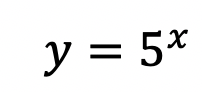, but it is only that, an Example! The exact pattern and exponential equation of the future progress of the virus is unknown! We mathematicians, can only measure what has already occurred and prepare/model for the future.  To make the virus spread less rapidly, it is our duty to stay home to slow the rate of this exponentially spreading virus as much as possible.

We want to #FlattenTheCurve a.k.a flatten the increasing exponential curve of new coronavirus cases that appear every day! Hopefully, this post brings some clarity to what’s going on in the world right now.  Even with mathematics, the true outcome of the virus may be unknown, but understanding why we are all at home in the first place and the positive impact it has is also important (and kind of cool).

Stay safe math friends and happy calculating! 🙂

Want to make math suck just a little bit less? Subscribe and follow us for FREE fun colorful math videos and lessons every week! 🙂

## Volume of a Cone: Geometry

The Voluminous “Vessel” at Hudson Yards

Calling all NYC dwellers! Have you seen the new structure at Hudson Yards?  A staircase to nowhere, this bee-hive like structure is for the true adventurists at heart; Clearly, I had to check it out!

Where does math come in here you say?  Well, during my exploration, I had to wonder (as am sure most people do) what is the volume of this almost cone-like structure? It seemed like the best way to estimate the volume here, was to use the formula for the volume of a cone!

## Volume of a Cone:

I estimated the volume by using the formula of a three-dimensional cone. (Not an exact measurement of the Vessel, but close enough!) .

We can find the radius and height based on the given information above.  Everything we need for our formula is right here!

Now that we have our information, let’s fill in our formula and calculate!

Extra Tip! Notice that we labeled the solution with feet cubed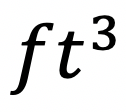, which is the short-handed way to write “feet cubed.”  Why feet cubed instead of feet squared? Or just plain old feet? When we use our formula we are multiplying three numbers all measured in feet:

All three values are measured in feet! –> Feet cubed ()